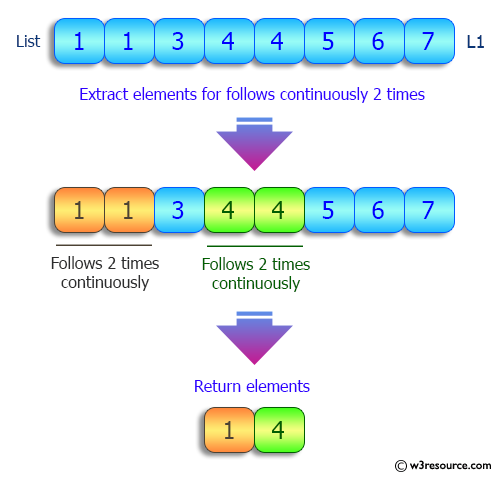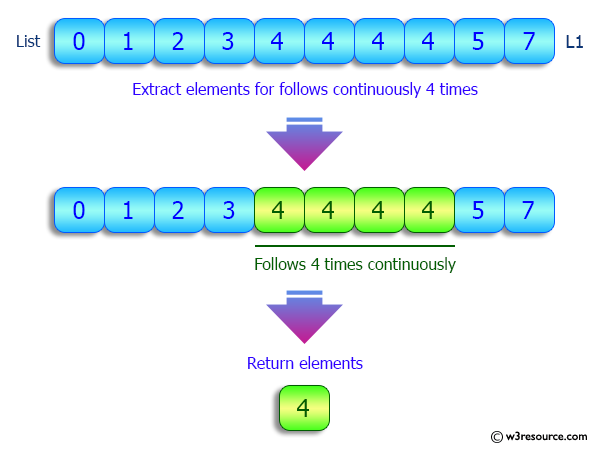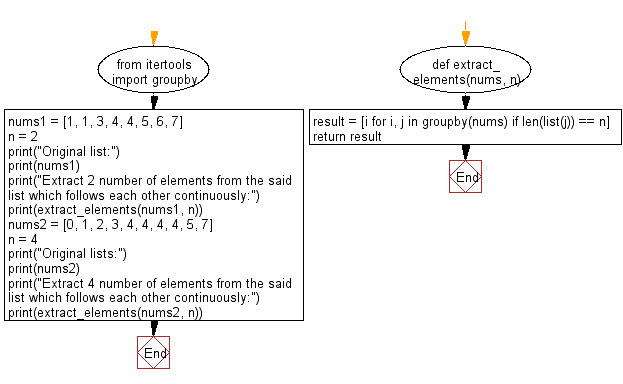﻿ Python: Extract specified number of elements from a given list, which follows each other continuously - w3resource# Python: Extract specified number of elements from a given list, which follows each other continuously

## Python List: Exercise - 103 with Solution

Write a Python program to extract specified number of elements from a given list, which follows each other continuously.

Sample Solution:

Python Code:

``````from itertools import groupby

def extract_elements(nums, n):
result = [i for i, j in groupby(nums) if len(list(j)) == n]
return result

nums1 = [1, 1, 3, 4, 4, 5, 6, 7]
n = 2
print("Original list:")
print(nums1)
print("Extract 2 number of elements from the said list which follows each other continuously:")
print(extract_elements(nums1, n))
nums2 = [0, 1, 2, 3, 4, 4, 4, 4, 5, 7]
n = 4
print("Original lists:")
print(nums2)
print("Extract 4 number of elements from the said list which follows each other continuously:")
print(extract_elements(nums2, n))
```
```

Sample Output:

```Original list:
[1, 1, 3, 4, 4, 5, 6, 7]
Extract 2 number of elements from the said list which follows each other continuously:
[1, 4]
Original lists:
[0, 1, 2, 3, 4, 4, 4, 4, 5, 7]
Extract 4 number of elements from the said list which follows each other continuously:

```

Pictorial Presentation:Flowchart:## Visualize Python code execution:

The following tool visualize what the computer is doing step-by-step as it executes the said program:

Python Code Editor:

Have another way to solve this solution? Contribute your code (and comments) through Disqus.

What is the difficulty level of this exercise?

Test your Python skills with w3resource's quiz

﻿

## Python: Tips of the Day

```print(2_000_000)
```2000000